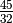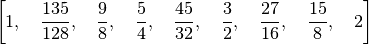# Number Theory¶

While this part of the package isn’t particularly fleshed out yet, there are a few number-theoretic functions for the analysis of scales.

## Odd Limits¶

PyTuning contains functions for finding the odd Limit for both intervals and scales.

We can define and interval – say,, and fine its odd-limit with the following:

from pytuning.number_theory import odd_limit

interval = sp.Rational(45,32)
limit = odd_limit(interval)


which yields and answer of 45.

One can also find the odd limit of an entire scale with the find_odd_limit_for_scale() function:

from pytuning.scales import create_euler_fokker_scale
from pytuning.number_theory import find_odd_limit_for_scale

scale = create_euler_fokker_scale([3,5],[3,1])
limit = find_odd_limit_for_scale(scale)


which yields 135. (Examining the scale:you will see that this is the largest odd number, and is found in the second degree.)

## Prime Limits¶

One can also compute prime limits for both scales and intervals. Extending the above example, one would assume that the Euler-Fokker scale would have a prime-limit of 5, since that’s the highest prime used in the generation, and in fact:

from pytuning.scales import create_euler_fokker_scale
from pytuning.number_theory import find_prime_limit_for_scale

scale = create_euler_fokker_scale([3,5],[3,1])
limit = find_prime_limit_for_scale(scale)


will return 5 as the limit.